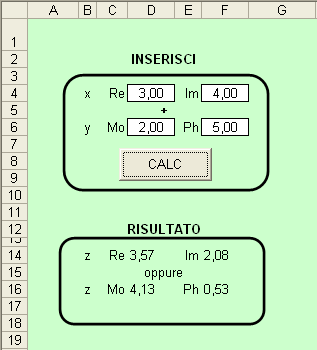Foglio1

 Private Sub CommandButton1_Click() Dim x As Complesso Dim y As Complesso Dim z As Complesso x.Re = Range("D4").Value x.Im = Range("F4").Value x = ReIm_to_MoPh(x) y.Mo = Range("D6").Value y.Ph = Range("F6").Value y = MoPh_to_ReIm(y) z = SommaComplessa(x, y) Range("D14").Value = z.Re Range("F14").Value = z.Im Range("D16").Value = z.Mo Range("F16").Value = z.Ph End Sub

Modulo1

 Type Complesso Re As Double Im As Double Mo As Double Ph As Double End Type Function ReIm_to_MoPh(a As Complesso) As Complesso a.Mo = Sqr(a.Re ^ 2 + a.Im ^ 2) If a.Re >= 0 Then If a.Re = 0 Then a.Re = 0.000000000000001 End If a.Ph = Atn(a.Im / a.Re) Else a.Ph = Atn(a.Im / a.Re) + Pi End If ReIm_to_MoPh = a End Function Function MoPh_to_ReIm(a As Complesso) As Complesso a.Re = a.Mo * Cos(a.Ph) a.Im = a.Mo * Sin(a.Ph) MoPh_to_ReIm = a End Function Function SommaComplessa(a As Complesso, b As Complesso) As Complesso Dim c As Complesso c.Re = a.Re + b.Re c.Im = a.Im + b.Im c.Mo = Sqr(c.Re ^ 2 + c.Im ^ 2) If c.Re >= 0 Then c.Ph = Atn(c.Im / c.Re) Else c.Ph = Atn(c.Im / c.Re) + Pi End If SommaComplessa = c End Function

SommaComplessa.xls